# GMAT Math : DSQ: Calculating the length of the diagonal of a quadrilateral

## Example Questions

### Example Question #91 : Geometry

Find the length of the diagonal of a square.

Statement 1:  The area is 1.

Statement 2:  The square is inscribed inside of a circle with all 4 corners touching the edge of the circle, and the circle has an area of 1.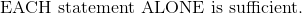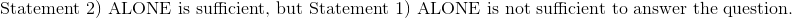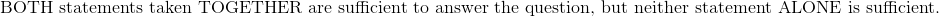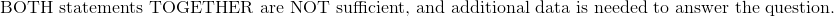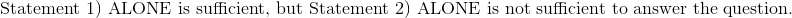Explanation:

Statement 1:  The area is 1.

Given the area of a square is sufficient to find the diagonal of the square.  The formula to find the side length of the square is: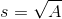The Pythagorean theorem can then be used to solve for the length of the diagonal.

Statement 2:  The square is inscribed inside of a circle with all 4 corners touching the edge of the circle, and the circle has an area of 1.

This statement is also sufficient to solve for the diagonal.  With all 4 corners of the square touching the circle, the diagonal of the square is simply the length from one of the square's opposing 90 degree angles to its opposite angle, which is the diameter of the circle.

Given the area of the circle, use the formula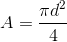to determine the diameter of the circle, which is also the diagonal of the square.

Therefore:### Example Question #92 : Geometry

Find the length of the diagonal of rectangle DEFT.

I) Side DE is 3 times the length of side TD.

II) The perimeter of the rectangle is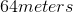.

Statement II is sufficient to answer the question, but statement I is not sufficient to answer the question.

Either statement is sufficient to answer the question.

Both statements are needed to answer the question.

Statement I is sufficient to answer the question, but statement II is not sufficient to answer the question.

Both statements are needed to answer the question.

Explanation:

Find the length of the diagonal of rectangle DEFT

I) Side DE is 3 times the length of side TD

II) The perimeter of the rectangle isWe need to find the diagonal of a rectangle. If we work back from there, we can see that to find the diagonal, we will need to the lengths of both sides of the rectangle.

I) Gives us the relationship between the two sets of sides: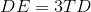II) Tells us the perimeter, for which we can write and equation: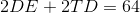Now, use the clue from I) to simplify our equation in II) to one variable: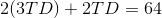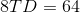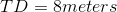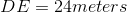So now that we have our side lengths, use Pythagorean Theorem to find the diagonal: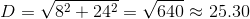### Example Question #93 : Geometry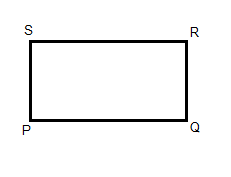For rectangle PQRS, the ratio of PQ to QR is 2 to 3. What is the length of SQ?

(1) The length of PS is 9.

(2) The length of SR is 6.

Statement (1) ALONE is sufficient, but statement (2) alone is not sufficient.

BOTH statements TOGETHER are sufficient, but NEITHER statement ALONE is sufficient.

Statement (2) ALONE is sufficient, but statement (1) alone is not sufficient.

EACH statement ALONE is sufficient.

Statements (1) and (2) TOGETHER are NOT sufficient.

EACH statement ALONE is sufficient.

Explanation:

With a ratio of sides of 2:3, statement 1 tells us PS and QR are 9 units long. Knowing the ratio we can determine both PQ and SR are 6. Since rectangles have four 90 degree angles, we can use the Pythagorean Theorem to solve for the length of SQ: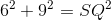. Therefore, statement 1 alone is sufficient.

With a ratio of sides of 2:3, statement 2 tells us PQ and SR are 6 units long. Knowing the ratio we can determine both PS and QR are 9 units long. Since rectangles have four 90 degree angles, we can use the Pythagorean Theorem to solve for the length of SQ:. Therefore, statement 2 alone is sufficient.

Therefore, the correct answer is  EACH statement ALONE is sufficient.

### Example Question #94 : Geometry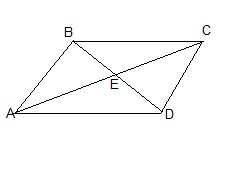For parallelogram ABCD, what is the length of AC?

(1) BD is 16.

(2) AE is 9.

EACH statement ALONE is sufficient.

BOTH statements TOGETHER are sufficient, but NEITHER statement ALONE is sufficient.

Statements (1) and (2) TOGETHER are NOT sufficient.

Statement (1) ALONE is sufficient, but statement (2) alone is not sufficient.

Statement (2) ALONE is sufficient, but statement (1) alone is not sufficient.

Statement (2) ALONE is sufficient, but statement (1) alone is not sufficient.

Explanation:

By definition, a parallelogram is a quadrilateral with 2 sets of parallel sides and diagonals that bisect each other.

From statement 1 we cannot determine the length of AC, since ABCD is only defined as a parallelogram and not necessarily a rectangle (which is a special parallelogram with equal diagonals). Therefore, statement 1 alone is not sufficient.

From statement 2, we can determine that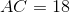since the definition of a parallelogram states the diagonals bisect each other. Therefore, Statement (2) ALONE is sufficient, but statement (1) alone is not sufficient.

### Example Question #95 : GeometryWhat is the length of PR?

(1) PQRS is a parallelogram

(2)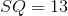BOTH statements TOGETHER are sufficient, but NEITHER statement ALONE is sufficient.

Statements (1) and (2) TOGETHER are NOT sufficient.

Statement (2) ALONE is sufficient, but statement (1) alone is not sufficient.

Statement (1) ALONE is sufficient, but statement (2) alone is not sufficient.

EACH statement ALONE is sufficient.

Statements (1) and (2) TOGETHER are NOT sufficient.

Explanation:

With the information from statement 1, we don't know the measurements of any parts of PQRS. Therefore,  Statement 1 alone is not sufficient.

With the information from statement 2, we know that, but since we have no confirmed definition of the shape of PQRS, we can't extrapolate the length of SQ to any other length.  Therefore, statement 2 alone is not sufficient.

Even if we look at both statements 1 and 2 together, quadrilateral PQRS looks like a rectangle, but we are only told it is a parallelogram. The figures on the GMAT are not drawn to scale, therefore your eyes cannot be trusted. No difinitive length of PR can be determined. Therefore, statements 1 and 2 together are not sufficient.

Therefore, the correct answer is Statements (1) and (2) TOGETHER are NOT sufficient.